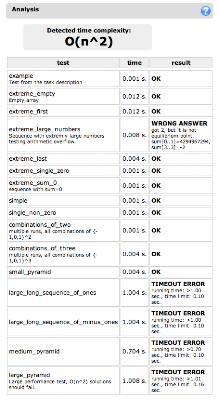# Equilibrium Index: A Solution For Equi Task | Codility

Codility's free Equi task is where coders practice and prepare before taking assessments. Here's our guide to getting the perfect equilibrium index score!

For programmers practicing their coding skills, or preparing to take a Codility assessment, we provide a series of free lessons, coding challenges and demo problems in our programmers' home

One of the most popular tasks is Equi and to date we have evaluated over 50,000 solutions to this problem!

The most popular programming language used for solving this problem is Java, although C#, PHP and C++ are also very popular:The problem description is very short:

The equilibrium index of a sequence is an index such that the sum of elements at lower indexes is equal to the sum of elements at higher indexes. For example, in a sequence A:

A=-7 A=1 A=5 A=2 A=-4 A=3 A=0

3 is an equilibrium index, because:

A+A+A=A+A+A

6 is also an equilibrium index, because:

A+A+A+A+A+A=0

(The sum of zero elements is zero) 7 is not an equilibrium index - because it is not a valid index of sequence A. If you still have doubts, here is a precise definition: The integer k is an equilibrium index of a sequence A,A..,A[n-1] if and only if 0<= kand sum(A[0..(k-1)])=sum(A[(k+1)..(n-1)]). Assume the sum of zero elements is equal to zero.

Write a function

```int equi(int A[], int n)
```

that, given a sequence, returns its equilibrium index (any) or -1 if no equilibrium index exists. Assume that the sequence may be very long.

The problem can be solved by using various approaches, the most common being simply to follow the equilibrium definition:

```int equi ( int A[], int n ) {
int k, m, lsum, rsum;
for(k = 0; k < n; ++k) {
lsum = 0; rsum = 0;
for(m = 0; m < k; ++m) lsum += A[m];
for(m = k + 1; m < n; ++m) rsum += A[m];
if (lsum == rsum) return k;
}
return -1;
}
```

Unfortunately, this approach has two disadvantages:

• it fails on large input data sets, since the time complexity is O(n2)
• it fails on large input values (for example if the input array contains values like MIN/MAX_INT) due to the arithmetic overflows

The solution analysis will detect such problems in submitted code:We can fix the first problem with a better algorithm, and the second problem with a better data-type (for example, using long long type instead of int for sum computations). The key observation for better running time is to update the left/right sums in constant time instead of recomputing them from the scratch.

```int equi(int arr[], int n) {
if (n==0) return -1;
long long sum = 0;
int i;
for(i=0;i<n;i++) sum+=(long long) arr[i];
long long sum_left = 0;
for(i=0;i<n;i++) {
long long sum_right = sum - sum_left - (long long) arr[i];
if (sum_left == sum_right) return i;
sum_left += (long long) arr[i];
}
return -1;
}
```

Using this solution, you can obtain a perfect score:What's next?

We have a live competition to write the shortest Equi solution - 54 bytes is smallest solution to date! If you're preparing for a Codility assessment, we offer two other demo problems which are free to take and can help you warm up:

Happy coding and good luck on your coding assessment